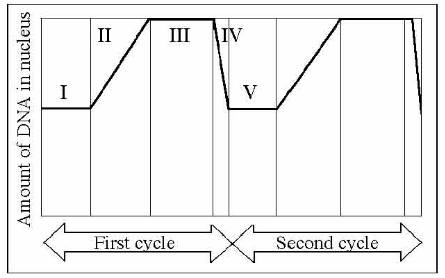# Problem: In the figure below, mitosis is represented by which numbered part(s) of the cycle?A) IB) IIC) IIID) IVE) V

###### Problem Details

In the figure below, mitosis is represented by which numbered part(s) of the cycle?

A) I

B) II

C) III

D) IV

E) VWhat scientific concept do you need to know in order to solve this problem?

Our tutors have indicated that to solve this problem you will need to apply the Introduction to the Cell Cycle concept. You can view video lessons to learn Introduction to the Cell Cycle. Or if you need more Introduction to the Cell Cycle practice, you can also practice Introduction to the Cell Cycle practice problems.

What is the difficulty of this problem?

Our tutors rated the difficulty ofIn the figure below, mitosis is represented by which numbere...as high difficulty.

How long does this problem take to solve?

Our expert Biology tutor, Kaitlyn took 7 minutes and 10 seconds to solve this problem. You can follow their steps in the video explanation above.

What professor is this problem relevant for?

Based on our data, we think this problem is relevant for Professor Yeargain's class at UCF.